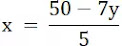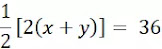# Pair of Linear Equations in Two VariablesExercise – 3.2

1. Form the pair of linear equations in the following problems and find their solutions graphically.

(i) 10 Students of Class x took part in a Mathematics quiz. If the number of girls is 4 more than the number of boys, find the number of boys and girls who took part in the quiz.

(ii) 5 pencils and 7 pens together cost ₹50, whereas 7 pencils and 5 pens together cost ₹46. Find the cost of one pencil and that of one pen.

Solution:

(i) Let the number of boys = y

Let the number of girls = x

Total number of students = 10

So, x + y = 10 ………………..(1)

Now, according to the question, the number of girls is 4 more than the number of boys,

x = y + 4 ……………….(2).(i)नानुसार

Both equations need three solutions to be expressed graphically.

From equation (1),

x + y = 10

x = 10 - y

 x 4 6 5 y 6 4 5

From equation (2),

x = y + 4

 x 5 3 7 y 1 -1 3

On looking at the graph, we find that the point of intersection of the equations is (7, 3) which is the common solution of the linear equations.

Hence, number of girls = 7 and number of boys = 3.

(ii) Let the cost of one pencil = x

Let the cost of a pen = y

According to the first condition,

So, 5x + 7y = 50 ………………..(1)

According to the second condition,

7x + 5y = 46 ……………….(2).(i)नानुसार

Both equations need three solutions to be expressed graphically.

From equation (1),

5x + 7y = 50On looking at the graph, we find that the point of intersection of the equations is (3, 5) which is the common solution of the linear equations.

Hence, cost of a pencil = ₹ 3 and cost of a pen = ₹ 5.

2. On comparing the ratios a1/a2, b1/b2 and c1/c2 find our whether the lines representing the following pairs of linear equations intersect at a point, are parallel or coincident:

(i)5x – 4y + 8 = 0

7x + 6y – 9 = 0

Solution:

a1 = 5, b1 = -4, c1 = 8

a2 = 7, b2 = 6, c2 = -9
Here,

Here,

Hence pair of linear equations are coincident.

(iii)6x - 3y + 10 = 0

2x - y + 9 = 0

Solution:

a1 = 6, b1 = -3, c1 = 10

a2 = 2, b2 = -1, c2 = 9

Hence, pair of linear equations are parallel.

3.  On comparing the ratios a1/a2, b1/b2 and c1/c2 find out whether the following pair of linear equations are consistent or inconsistent:

(i)3X + 2Y = 5, 2X - 3Y = 7

Solution:

a1 = 3, b1 = 2, c1 = 5

a2 = 2, b2 = -3, c2 = 7
Here,

Hence, the pair of linear equations Intersect at one point and the pair of linear equations are consistent.

(ii)2X - 3Y = 8, 4X - 6Y = 9

Solution:

a1 = 2, b1 = -3, c1 = 8

a2 = 4, b2 = -6, c2 = 9
Here,

Hence, the pair of linear equations are parallel and the pair of linear equations are inconsistent.

(iii) (3/2)x + (5/3)y = 7, 9x – 10y = 14

Solution:

9x + 10y = 42, 9x – 10y = 14

a1 = 9, b1 = 10, c1 = 42

a2 = 9, b2 = -10, c2 = 14
Here,

Hence, the pair of linear equations intersect at one point and the pair of linear equations is consistent.

(iv) 5x - 3y = 11, -10x + 6y = -22

Solution:

a1 = 5, b1 = -3, c1 = 11

a2 = -10, b2 = 6, c2 = -22

Hence, the pair of linear equations are consistent.

(v) (4/3)x + 2y = 8, 2x + 3y = 12

Solution:

a1 = 4/3, b1 = 2, c1 = 8

a2 = 2, b2 = 3, c2 = 12

Hence, the pair of linear equations are consistent.

4. Which of the following pairs of linear equations are consistent/inconsistent? If consistent obtain the solution graphically:

(i) x + y =5, 2x + 2y = 10

Solution:

a1 = 1, b1 = 1, c1 = 5

a2 = 2, b2 = 2, c2 = 10
Here,

Hence, the pair of linear equations are consistent.

Both equations need three solutions to be expressed graphically.

From equation (1),

x + y =5

x = 5 – y

 x 4 3 2 y 1 2 3

From equation (2),

x = (10-2y)/2

 x 4 3 2 y 1 2 3

Hence, the pair of linear equations are consistent.

Both equations need three solutions to be expressed graphically.

From equation (1),

x = (6 – y)/2

 x 0 1 2 y 6 4 2

From equation (2),

x = (4 + 2y)/4

 x 1 2 3 y 0 2 4

Here,

Hence, the pair of linear equations are inconsistent.

5. Half the perimeter of a rectangular garden, whose length is 4 m more than its width, is 36 m. Find the dimensions of the garden.

Solution:

Let the width of the rectangular garden = x m

Let the length of the rectangular garden = y m

According to Question,

Half of the perimeter = 36 m

Perimeter/2 = 36x + y = 36…………………(i)

The length of the garden is 4 m more than the width,

so

y – x = 4…………………(ii)

Both equations need three solutions to be expressed graphically.

From equation (1),

x = 36 – y

 x 0 36 20 y 36 0 16

From equation (2),

y = x + 4

 x 0 -4 5 y 4 0 9

The point of intersection of the equations is (16, 20). Hence, the length of the garden is 20 m and the width is 16 m.

6. Given the linear equation 2x + 3y – 8 = 0 write another linear equation in two variables such that the geometrical representation of the pair so formed is:

(i) intersecting lines

Solution:

The linear equation 2x + 3y – 8 = 0 intersects the linear equation x + 3y – 10 = 0 because,

a1 = 2, b1 = 3, c1 = -8

a2 = 1, b2 = 3, c2 = -10

(ii) parallel lines

Solution:

The linear equation 2x + 3y – 8 = 0 is parallel to the linear equation 4x + 6y + 5 = 0 because,

(iii) coincident lines

Solution:

The linear equation 2x + 3y – 8 = 0 coincides with the linear equation 4x + 6y – 16 = 0 because,

7. Draw the graphs of the equations x – y + 1 = 0 and 3x + 2y – 12 = 0. Determine the coordinates of the vertices of the triangle formed by these lines and the x axis, and shade the triangular region.

Solution:

x – y + 1 = 0…………..(i)

3x + 2y – 12 = 0………..(ii)

Both equations need three solutions to be expressed graphically.

From equation (1),

y = x + 1

 x 0 -1 2 y 1 0 3

From equation (1),

3x + 2y – 12 = 0

 x 0 2 4 y 6 3 0

The coordinates of the vertices of the triangle formed by these lines and the x-axis are (-1, 0), (2, 3) and (4, 0).